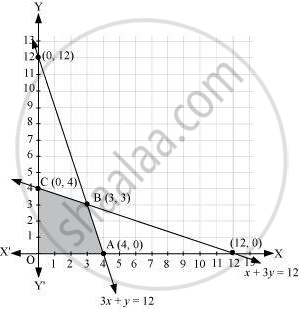# A manufacturer produces nuts and bolts. It takes 1 hour of work on machine A and 3 hours on machine B to produce a package of nuts. - Mathematics

Sum

A manufacturer produces nuts and bolts. It takes 1 hour of work on machine A and 3 hours on machine B to produce a package of nuts. It takes 3 hours on machine A and 1 hour on machine B to produce a package of bolts. He earns a profit of ₹ 35 per package of nuts and ₹ 14 per package of bolts. How many packages of each should be produced each day so as to maximize his profit, if he operates each machine for almost 12 hours a day? convert it into an LPP and solve graphically.

#### Solution

Let the manufacturer produce x packages of nuts and y packages of bolts. Therefore,

x ≥ 0 and y ≥ 0

The given information can be compiled in a table as follows.

 Nuts Bolts Availability Machine A (h) 1 3 12 Machine B (h) 3 1 12

The profit on a package of nuts is Rs 35 and on a package of bolts is Rs 14. Therefore, the constraints are

x + 3y ≤ 12
3x + y ≤ 12

Total profit, Z = 35x + 14y

The mathematical formulation of the given problem is

Maximise Z = 35x + 14y    ...… (1)

subject to the constraints,
x + 3y ≤ 12  ....… (2)

3x + y ≤ 12  ….... (3)

x, y ≥ 0   …........ (4)

The feasible region determined by the system of constraints is as follows.The corner points are A(4, 0), B(3, 3), and C(0, 4).

The values of Z at these corner points are as follows.

 Corner point Z = 35 x + 14 y O(0, 0) 0 A(4, 0) 140 B(3, 3) 147  → Maximum C(0, 4) 56

The maximum value of Z is Rs 147 at (3, 3).

Thus, 3 packages of nuts and 3 packages of bolts should be produced each day to get the maximum profit of Rs 147.

Concept: Different Types of Linear Programming Problems
Is there an error in this question or solution?